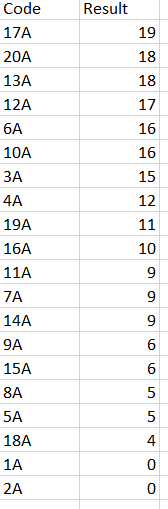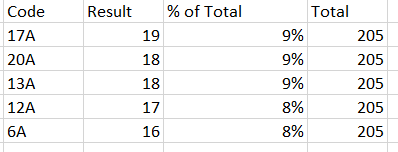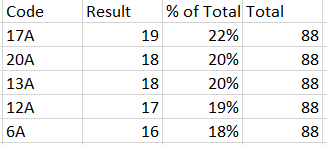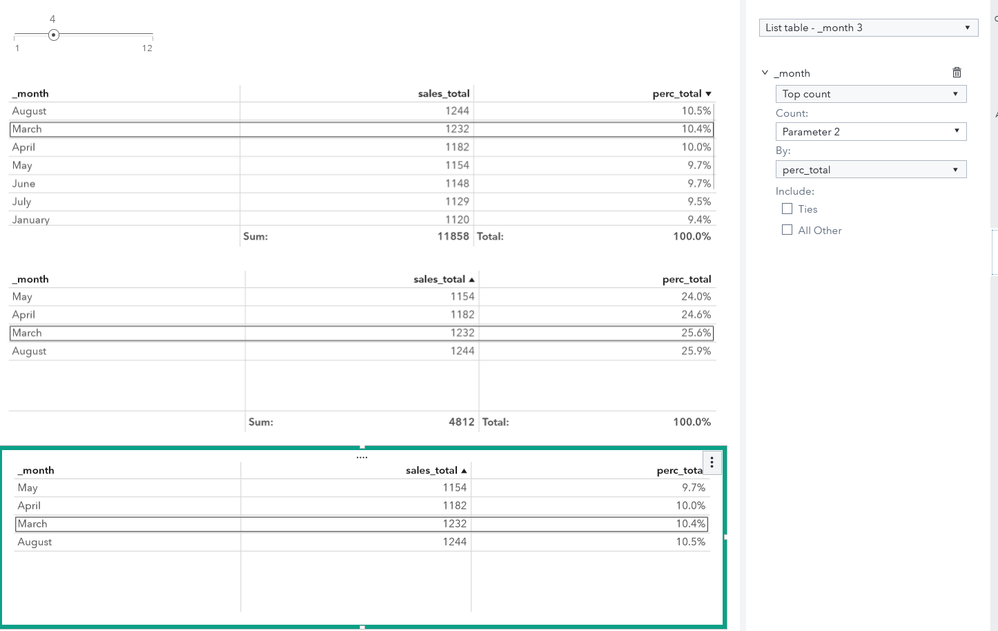## calculate % column total?

Hi all,

Currently, I am trying to show a top 5 rank in VA within a list table. Showing just the top 5 rank in itself works fine. However, I have now been asked to show how much % of the total a score is.

For example, my data looks like the following:I would then like to show the results in a top 5, like this:However, what currently happens is that VA only calculates and shows the total, based on the visible rows, like this:As such, I was wondering if it's possible to calculate a % of the total within a column in VA version 7.5, while only showing a top 5 rank?

4 REPLIES 4

## Re: calculate % column total?

If you include the All Other in the ranking, does it rank the percentages as you would like?

## Re: calculate % column total?

One possible solution is to create a aggregated table and include the calculated percentage as a variable.
With the aggregated table the variable becomes static in the scope of this table.
Now you can rank based on the top5 and show the share correctly. The last object builds upon the aggregated table.I present you an alternative as well:
There is a pareto example out there in the communities which uses job execution for that purpose.

## Re: calculate % column total?

I would add the "All other" ranking.

When you create an aggregated table, you won't be able to calculate correct percentages when you start adding filters to your report or when change the variables in your rows.

Been there, done that 😉

## Re: calculate % column total?

If I do not understand wrongly your concern, you can achieve updating the aggregated table correctly applying parameters in the filter of the aggregated table.

this should keep them synchronized.

Discussion stats
• 4 replies
• 409 views
• 0 likes
• 4 in conversation